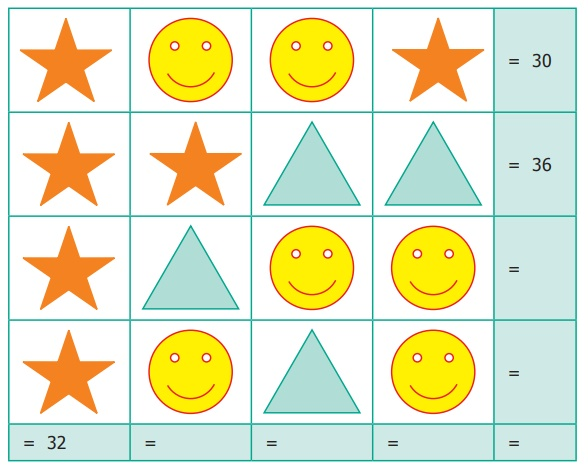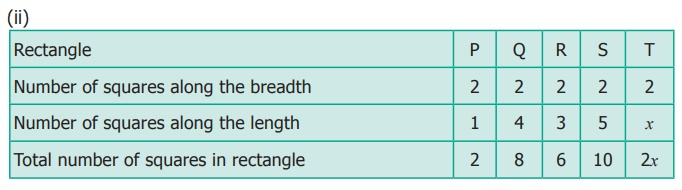Home | | Maths 6th Std | Exercise 2.3

# Exercise 2.3

6th Maths : Term 1 Unit 2 : Introduction To Algebra : Text Book Back Exercises Questions with Answers, Solution

Exercise 2.3

Miscellaneous Practice Problems

1. Complete the following pattern.

9 – 1 =

98 – 21 =

987 – 32 =

9876 − 432 =

98765 − 54321 =

What comes next?

9 – 1 = 8

98 − 21 =77

987 − 321 = 666

9876 − 4321 = 5555

98765 − 54321 = 44444

987654 − 654321 = 3333333

2. A piece of wire is ‘12s’ cm long. What will be the length of the side, if it is formed as

(i) an equilateral triangle

(ii) a square?

Solution:

(i) equilateral triangle = 3 equal sides

A piece wire [circumference] = 12s

one side length = 12s / 3

= 4s

(ii) Square = 4 equal sides

Circumference = 12s

One side length = 12s / 4

= 3s

3. Identify the value of the shapes and figures in the table given below and verify their addition horizontally and vertically.4. The table given below shows the results of the matches played by 8 teams in a Kabaddi championship tournament.Find the value of all the variables in the table given above.

Solution:

k = 8 – 5 = 3

a = 7 + 2 = 9

x = 8 – 4 = 4

m = 7 – 6 = 1

b = 9 – 3 = 6

y = 6 + 3 = 9

n = 4 + 6 = 10

c = 10 – 6 = 4

Challenging Problems

5. Gopal is 8 years younger to Karnan. If the sum of their ages is 30, how old is Karnan?

Solution:

Age of Karnan = x

Age of Gopal = y

Gopal is 8 years younger to Karnan

i.e., y = x − 8

sum of their age = 30

x + y = 30

x + (x − 8) = 30

x + x − 8 = 30

2x − 8 = 30

2x = 30 + 8

2x = 38

x = 38 / 2 = 19

Age of Karnan is ‘19’ years.

6. The rectangles made of identical square blocks with varying lengths but having only two square blocks as width are given below.(i) How many small size squares are there in each of the rectangles P, Q, R and S?

P = 2, Q = 8, R = 6, S= 10

(ii) Fill in the boxes.7. Find the variables from the clues given below and solve the cross−word puzzle.Across

x + 40 gives 100

7 reduced from t gives 31

z is 5 added 5 times

v is the whole number zero plus number of days in a year

k is 24 added to 25

u is 2 added to two times 11 gives the number of hours in a day

a is 20 more to 40

s minus 1 gives 246 is the number of letters in Tamil language

Down

x is 1005 multiplied by 6

t ÷ 7 = 5

p is the predecessor of first 3 digit number

z is the number of weeks in a year (digits reversed)

k is 11 times 4

u is product of 23 and 9

a is 4 added to the product of 12 and 5

m is the successor of 9

Exercise 2.3

1) 8; 77; 666; 5555; 44444; 333333

2) (i) 4s (ii) 3s

3)4) k = 3; m = 1; n = 10; a = 9; b = 6; c = 4; x = 4; y = 9.

5) 19

6) (i) P=2; Q=8; R=6; S=107)Tags : Questions with Answers, Solution | Introduction to Algebra | Term 1 Chapter 2 | 6th Maths , 6th Maths : Term 1 Unit 2 : Introduction To Algebra
Study Material, Lecturing Notes, Assignment, Reference, Wiki description explanation, brief detail
6th Maths : Term 1 Unit 2 : Introduction To Algebra : Exercise 2.3 | Questions with Answers, Solution | Introduction to Algebra | Term 1 Chapter 2 | 6th Maths